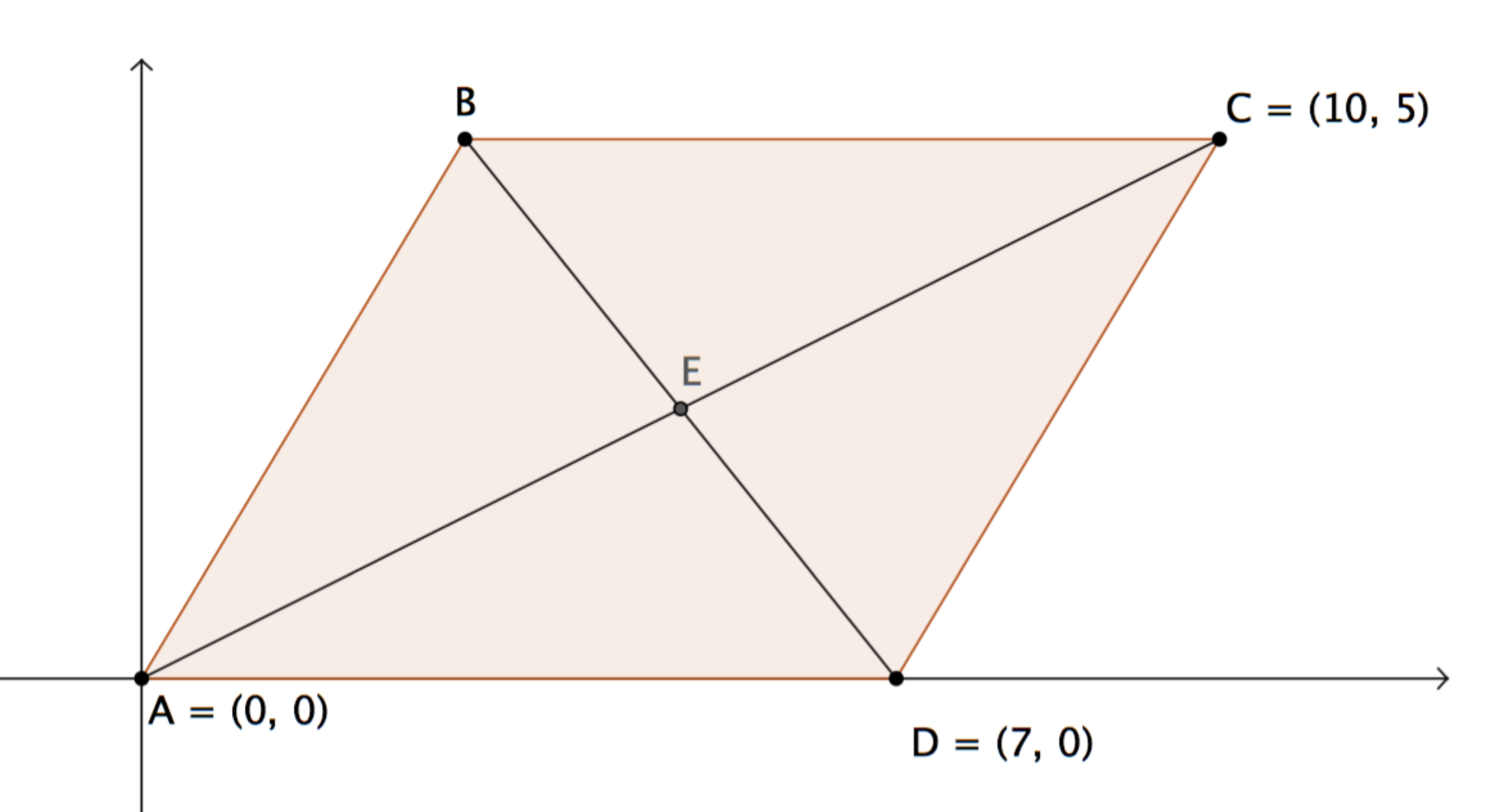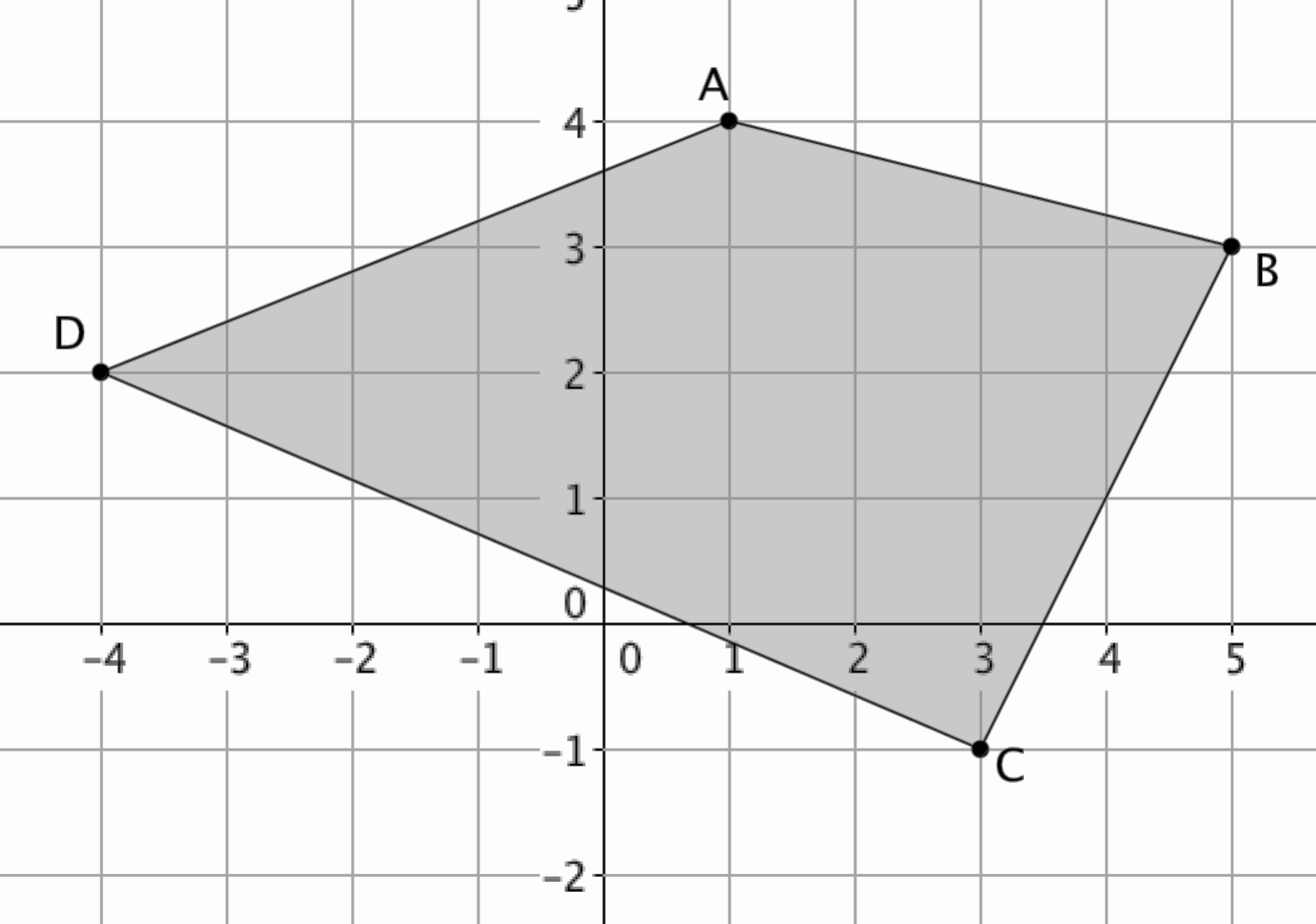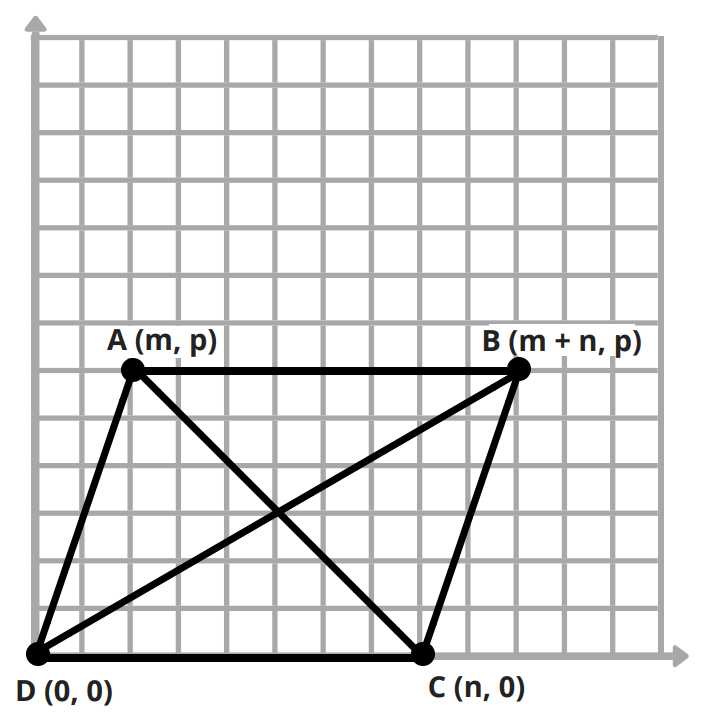# Polygons and Algebraic Relationships

## Objective

Algebraically verify diagonal relationships in quadrilaterals and parallelograms.

## Common Core Standards

### Core Standards

?

• G.CO.C.11 — Prove theorems about parallelograms. Theorems include: opposite sides are congruent, opposite angles are congruent, the diagonals of a parallelogram bisect each other, and conversely, rectangles are parallelograms with congruent diagonals.

• G.GPE.B.4 — Use coordinates to prove simple geometric theorems algebraically. For example, prove or disprove that a figure defined by four given points in the coordinate plane is a rectangle; prove or disprove that the point (1, √3) lies on the circle centered at the origin and containing the point (0, 2).

?

• G.CO.C.9

## Criteria for Success

?

1. Verify algebraically that when diagonals bisect each other in a quadrilateral, that quadrilateral is a parallelogram.
2. Describe characteristics of quadrilaterals, and more specifically trapezoids and parallelograms, with respect to midsegments and diagonals. Verify algebraically.
3. Identify quadrilaterals that have perpendicular diagonals, congruent diagonals, or proportional diagonals through algebraic reasoning.
4. Identify missing coordinate points in parallelograms, trapezoids, and rectangles using algebraic reasoning.

## Tips for Teachers

?

• This lesson connects to Unit 2 Lessons 17 and 18 because it deals with properties of quadrilaterals and parallelograms that were developed off the coordinate plane using proof. This lesson will expand the knowledge of these properties and work with them on the coordinate plane.
• Students may need to review properties of parallelograms, particularly from Unit 2 Lesson 18 before they can fully access this lesson. It is recommended to spend time outside of class building this specific skill.

## Anchor Problems

?

### Problem 1

Below is parallelogram ${ABCD}$.

$E$ is the midpoint of diagonal ${\overline {AC}}$.

Prove that $\overline{BE}\cong \overline{ED}$.### Problem 2

Find the midpoints of each of the sides of the quadrilateral below to create a new quadrilateral with the midpoints as the vertices.What do you notice about the quadrilateral formed by these midpoints? We call this the inscribed quadrilateral.

#### References

Illustrative Mathematics A Midpoint Miracle

A Midpoint Miracle, accessed on June 4, 2018, 1:04 p.m., is licensed by Illustrative Mathematics under either the CC BY 4.0 or CC BY-NC-SA 4.0. For further information, contact Illustrative Mathematics.

Modified by Fishtank Learning, Inc.

### Problem 3

What particular shape has perpendicular and congruent diagonals?

## Problem Set

?

The following resources include problems and activities aligned to the objective of the lesson that can be used to create your own problem set.

• Include problems related to Anchor Problem #1, such as a question using Geometry – 6.10 IP created on GeoGebra
• Include problems where students need to identify statements about diagonals as true or false and explain their reasoning, such as “the diagonals of the trapezoid intersect at the same point as the segment that connects the midpoints of the two bases” or “the diagonals of the trapezoid intersect at the same point as the segment that connects the midpoints of the legs of the trapezoid.”